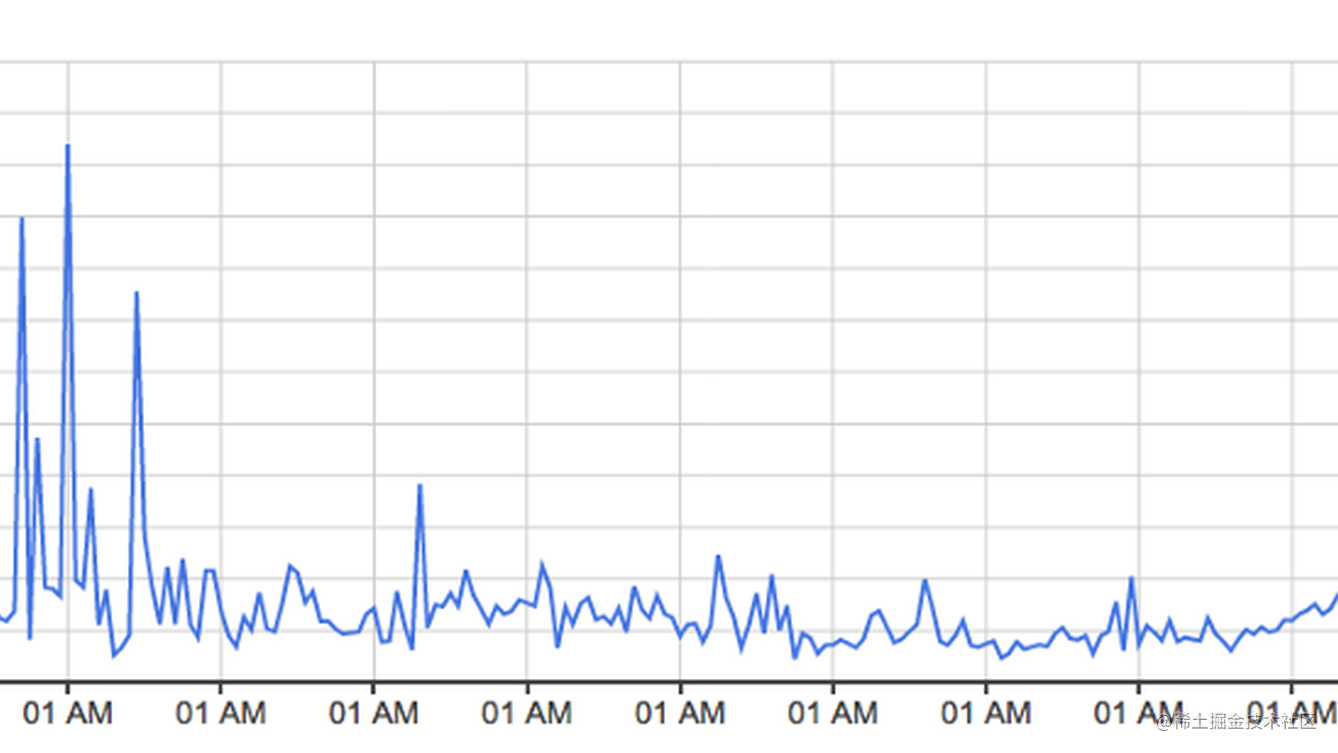[译] 使用 python 分析 14 亿条数据使用 python 分析 14 亿条数据

使用 pytubes，numpy 和 matplotlib

挑战

1-gram 的数据集在硬盘上可以展开成为 27 Gb 的数据，这在读入 python 时是一个很大的数据量级。Python可以轻易地一次性地处理千兆的数据，但是当数据是损坏的和已加工的，速度就会变慢而且内存效率也会变低。

1-gram 的数据是以 tab 键分割的形式储存在文件中，看起来如下：

Python 1587 4 2
Python 1621 1 1
Python 1651 2 2
Python 1659 1 1

1. Word
2. Year of Publication
3. Total number of times the word was seen
4. Total number of books containing the word

1. 这个单词是我们感兴趣的？
2. 发布的年份
3. 单词使用的总次数

import tubes

WORD = "Python"

# Set up the data load pipeline
one_grams_tube = (tubes.Each(FILES)
.split()
.multi(lambda row: (
row.get(0).equals(WORD.encode('utf-8')),
row.get(1).to(int),
row.get(2).to(int)
))
)

# 将数据读入一个 numpy 数组。通过设置一个大概的精准度
# 预估行数，pytubes 优化分配模式
# fields=True 这里是冗余的，但是确保了返回的 ndarray
# 使用字段，而不是一个单独的多维数组 one_grams = one_grams_tube.ndarray(estimated_rows=500_000_000, fields=True)

╒═══════════╤════════╤═════════╕
│   Is_Word │   Year │   Count │
╞═══════════╪════════╪═════════╡
│         0 │   1799 │       2 │
├───────────┼────────┼─────────┤
│         0 │   1804 │       1 │
├───────────┼────────┼─────────┤
│         0 │   1805 │       1 │
├───────────┼────────┼─────────┤
│         0 │   1811 │       1 │
├───────────┼────────┼─────────┤
│         0 │   1820 │     ... │
╘═══════════╧════════╧═════════╛

每一年的单词总使用量

last_year = 2008
YEAR_COL = '1'
COUNT_COL = '2'

year_totals, bins = np.histogram(
one_grams[YEAR_COL],
density=False,
range=(0, last_year+1),
bins=last_year + 1,
weights=one_grams[COUNT_COL]
)

one_grams_tube = (tubes.Each(FILES)
.split()
.skip_unless(lambda row: row.get(1).to(int).gt(1799))
.multi(lambda row: (
row.get(0).equals(word.encode('utf-8')),
row.get(1).to(int),
row.get(2).to(int)
))
)

Python 在每年的占比百分数

# 找到匹配的行 (column 是 Ture)
word_rows = one_grams[IS_WORD_COL]
# 创建一个空数组来保存每年占比百分数的值
word_counts = np.zeros(last_year+1)
# 迭代至每条匹配的数据 （匹配一个单词时，应该只有几千行数据）
for _, year, count in one_grams[word_rows]:
# 设置相关的 word_counts 行为计算后的数值
word_counts[year] += (100*count) / year_totals[year]

• 人们是如何将 Python 当做动词使用的？
• ‘Python’ 的计算总量是否包含 ‘Python_VERB’？等

语言战争

1. 只有首字母大写的名字形式能被匹配（Python，不是 python）
2. 每一个语言的提及总数已经被转换到了从 1800 年到 1960 年的百分比平均数，考虑到 Pascal 在 1970 年第一次被提及，这应该有一个合理的基准线。